Imagination is more important than knowledge... Albert Einstein

Guess is more important than calculation --- Knowhowacademy.com

Length - Pythagorean Theorem

For ACT
Number of Total Problems: 28.
FOR PRINT ::: (Book)

 Problem Num : 11 Type: None Section:Length Theme:None Adjustment# : 19 Difficulty: 1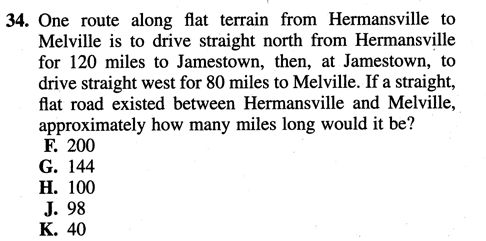Category Pythagorean Theorem
Analysis Can you see the right triangle?

 Problem Num : 12 Type: None Section:Length Theme:None Adjustment# : 19 Difficulty: 1Category Pythagorean Theorem
Analysis

 Problem Num : 13 Type: None Section:Length Theme:None Adjustment# : 21 Difficulty: 1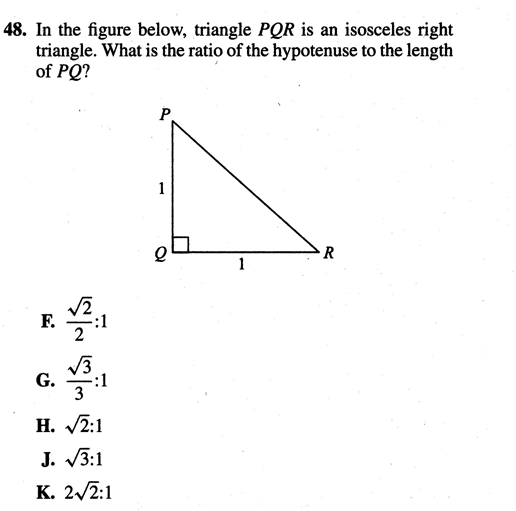Category Pythagorean Theorem
Analysis

 Problem Num : 14 Type: None Section:Length Theme:None Adjustment# : 22 Difficulty: 1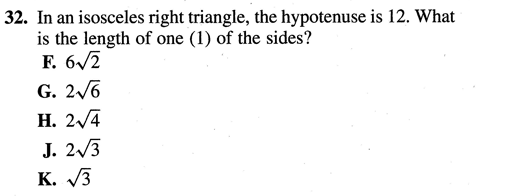Category Pythagorean Theorem
Analysis

 Problem Num : 15 Type: None Section:Length Theme:None Adjustment# : 22 Difficulty: 1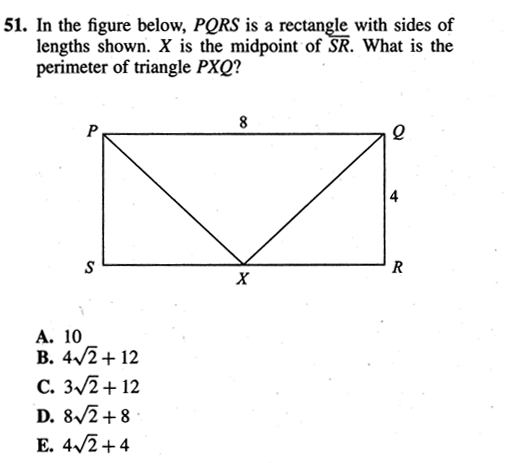Category Pythagorean Theorem
Analysis

 Problem Num : 16 Type: None Section:Length Theme:None Adjustment# : 24 Difficulty: 1Category Pythagorean Theorem
Analysis There are several ways to express the isosceles triangle.

 Problem Num : 17 Type: None Section:Length Theme:None Adjustment# : 27 Difficulty: 1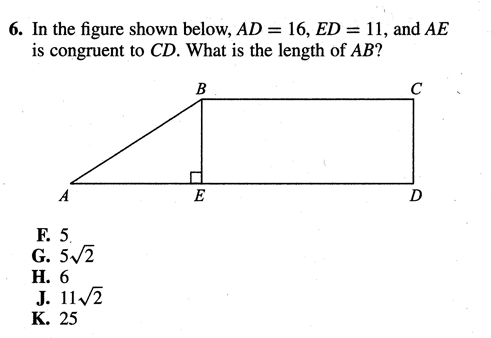Category Pythagorean Theorem
Analysis 1-1-?

 Problem Num : 18 Type: None Section:Length Theme:None Adjustment# : 30 Difficulty: 1Category Pythagorean Theorem
Analysis

 Problem Num : 19 Type: None Section:Length Theme:None Adjustment# : 32 Difficulty: 1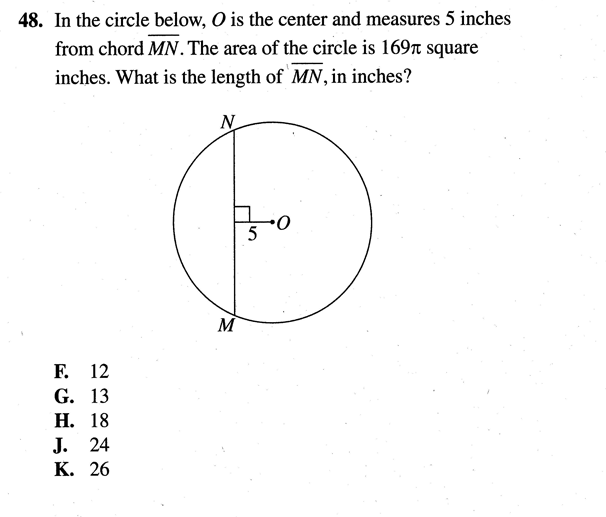Category Pythagorean Theorem
Analysis

 Problem Num : 20 Type: None Section:Length Theme:None Adjustment# : 32 Difficulty: 1Category Pythagorean Theorem
Analysis